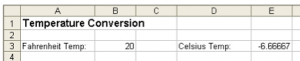# Temp

The local university’s Meteorology department wants to use a worksheet to convert Fahrenheit temperatures to the equivalent Celsius temperatures.

a) Create a new workbook.

b) Enter the data and apply formatting as shown below. In cell E3 enter the formula =5/9*(B3–32) to convert the Fahrenheit temperature stored in cell B3 to degrees Celsius:c) Save the workbook naming it Temp Conversion.

d) Format cell E3 to display 0 decimal places.

e) Apply conditional formatting to cells B3 and E3 so that negative values will be displayed in a red font color.

f) Enter the following Fahrenheit temperatures in cell B3, one at a time: 0, 32, and 80. What Celsius temperature does each of these convert to?

g) In row 5, have the worksheet convert temperatures from a Celsius temperature entered in cell B5 to a Fahrenheit temperature displayed in cell E5. Use 26 for the Celsius tem- perature. Include appropriate labels. The formula needed for converting from degrees Celsius to Fahrenheit is =9/5*B5+32. Display the result with 0 decimal places. Change the column widths as necessary so that all the data is displayed entirely.

h) Enter the following Celsius temperatures in cell B5, one at a time: 0, 12, and –21. What Fahrenheit temperature does each of these convert to?

i) In cell D1, add a link to a Web site, such as www.weather.com, where users could access current temperature data.

j) Create a header that right aligns your name and a footer that center aligns the current date. Add gridlines and row and column headings.

k) Save the modified Temp Conversion and print a copy.

l) Display the formulas in the cells instead of values. Change the column widths as necessary so that the formulas are completely displayed. Print a copy.